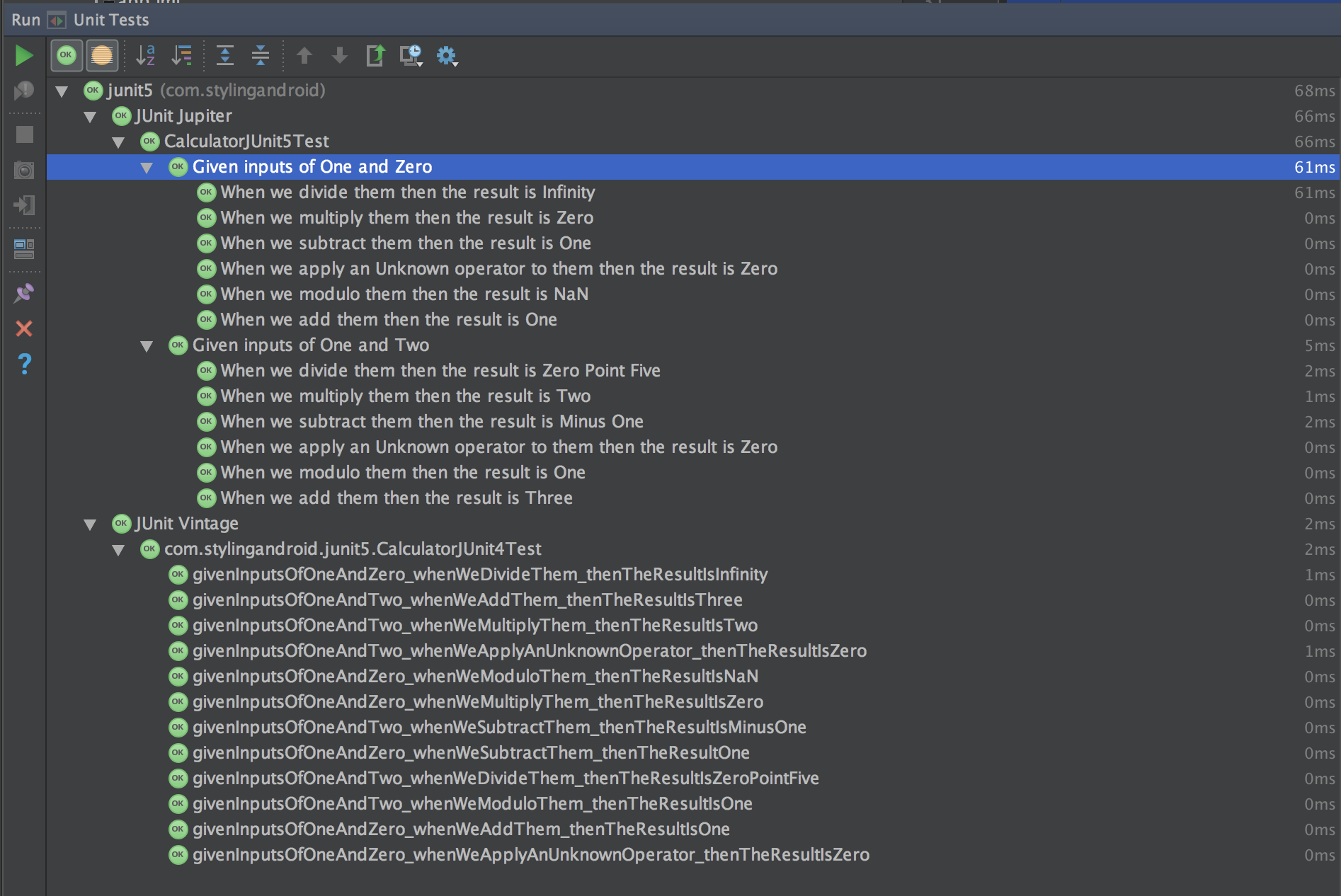# JUnit 5: Nested Tests

JUnit 5 was formally released in July 2016 and is quite a major evolution to JUnit 4 which has been the standard unit testing framework since Android first appeared on the scene. There are some quite significant changes and getting things set up, and then getting the best out of JUnit 5 can require a little effort, but the results are well worth it. In this loosely-coupled series we’ll explore a few distinct aspects of JUnit 5 and look at how we can get the best out of it. In this article we’ll look at some of the new features in JUnit 5 and see how they can simplify our tests.Previously we looked at how we can use the `@DisplayName` annotation to improve the readability of our test reports, however they are still pretty verbose and there is much boilerplate. In this article we’ll take a look at how we can use Nested Tests to begin to address this.

If we look at the current JUnit 5 test suite it should be quite obvious that in each individual test we define the two input values. Given that we have a total of twelve tests in the suite, but we only use two different sets of input values we should already be getting something of a smell that we should be looking to remove some of this duplication. Actually the first input value, named `input1`, does not change at all, so we should already be thinking of making this a fixed value. But the second input value, named `input2`, is a bit more tricky because it has a value of `2` for one half of the tests, and a value of `0` for the other half. Once of the new features of JUnit 5 which can help us with this is NestedTests.

A Nested Test is actually just an inner class. The only thing that we need to do is correctly annotate it so that it is recognised as a NestedTest. Within each inner class we can have localised state, and a set of tests. So it is an ideal way of grouping a set of tests which rely on a specific start state of the system under test. In other words, for all test which have the same “`Given...`” clause of the “`Given...When...Then...`” structure, then we can standardise that within a specific nested test.

In our case half of the tests have inputs of One and Two, and the other half have inputs of One and Zero. We can create two NestedTests to cover these two initial states – each Nested Test contains the six actual tests:

```public class CalculatorJUnit5Test {

private Calculator calculator;
final float input1 = 1;

@BeforeEach
void setup() {
calculator = new Calculator();
}

@Nested
@DisplayName("Given inputs of One and Two")
class oneAndTwo {
final float input2 = 2;

@Test
@DisplayName("When we add them Then the result is Three")
void plus() {
float result = calculator.calculate(input1, input2, Operator.PLUS);

assertThat(result).isEqualTo(3f);
}
.
.
.
}

@Nested
@DisplayName("Given inputs of One and Zero")
class oneAndZero {
final float input2 = 0;

@Test
@DisplayName("When we add them Then the result is One")
void plus() {
float result = calculator.calculate(input1, input2, Operator.PLUS);

assertThat(result).isEqualTo(1f);
}
.
.
.
}
}```

There is one really important thing in here – note how we are breaking up the `@DisplayName` annotations? Because the “`Given...`” state is now constant for each of the `@Nested` inner classes, we define this part of the `@DisplayName` with the `@Nested` inner class. Each of the `@DisplayName` annotations for individual tests now only need to contain the “`When...Then...`“.

This actually has an effect of the test report that gets generated:Now we actually get the test reports within a specific `@Nested` inner class listed within the parent. This actually improves the readability still further because it is actually grouping all of the tests for a given starting state together.

If we now look at the full JUnit5 test suite (which I won’t include here for space reasons) we have reduced things down from the previous 149 lines to 128. Being able to group tests for a given starting state of the system under test is a really useful thing to be able to do, particularly in cases where the system under test is rather more complicated than our simple calculator example. In particular it should make our tests a little more robust because we are not duplicating this state setup for each individual test – which smacks of copy & paste programming.

However, in our case there is still much of each individual test which is identical to all of the others, and in the next article in this series we’ll take a look at a way that we can further reduce the boilerplate when we have a set of largely identical tests.1.Thomas Keller says: